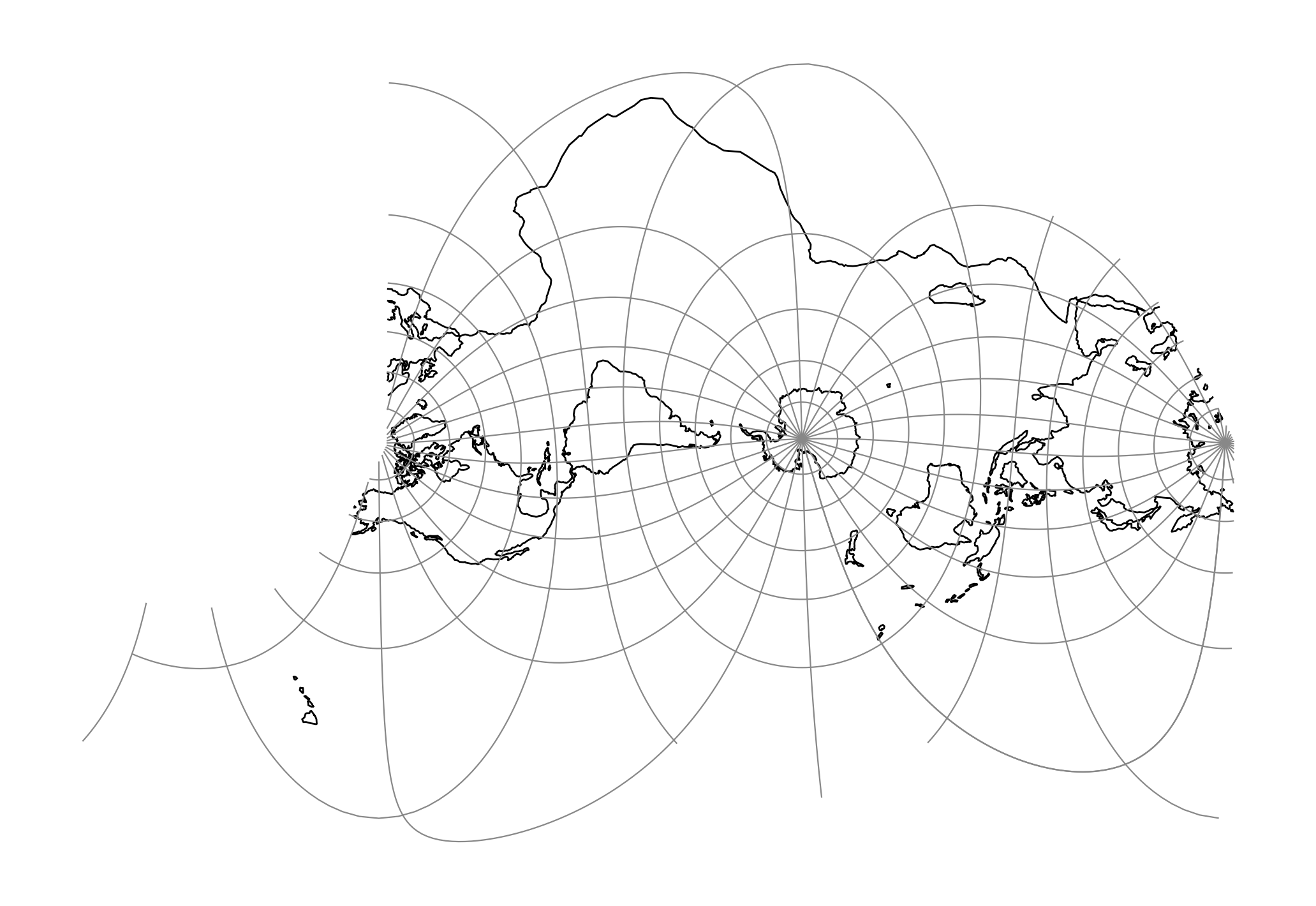# Space oblique for LANDSAT¶proj-string: +proj=lsat +ellps=GRS80 +lat_1=-60 +lat_2=60 +lsat=2 +path=2

## Parameters¶

### Required¶

+lsat=<value>

Landsat satellite used for the projection. Value between 1 and 5.

+path=<value>

Selected path of satellite. Value between 1 and 253 when +lsat is set to 1,2 or 3, otherwise valid input is between 1 and 233.

### Optional¶

+lon_0=<value>

Longitude of projection center.

Defaults to 0.0.

+ellps=<value>

See proj -le for a list of available ellipsoids.

Defaults to “GRS80”.

+R=<value>

Radius of the sphere given in meters. If used in conjunction with +ellps +R takes precedence.

+x_0=<value>

False easting.

Defaults to 0.0.

+y_0=<value>

False northing.

Defaults to 0.0.